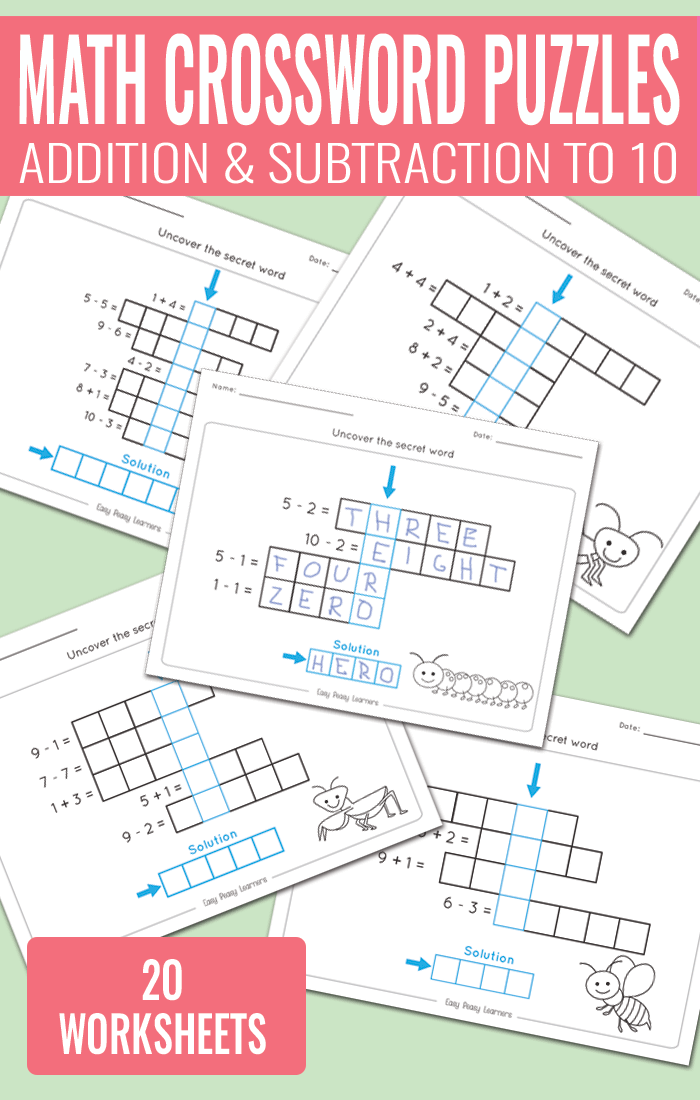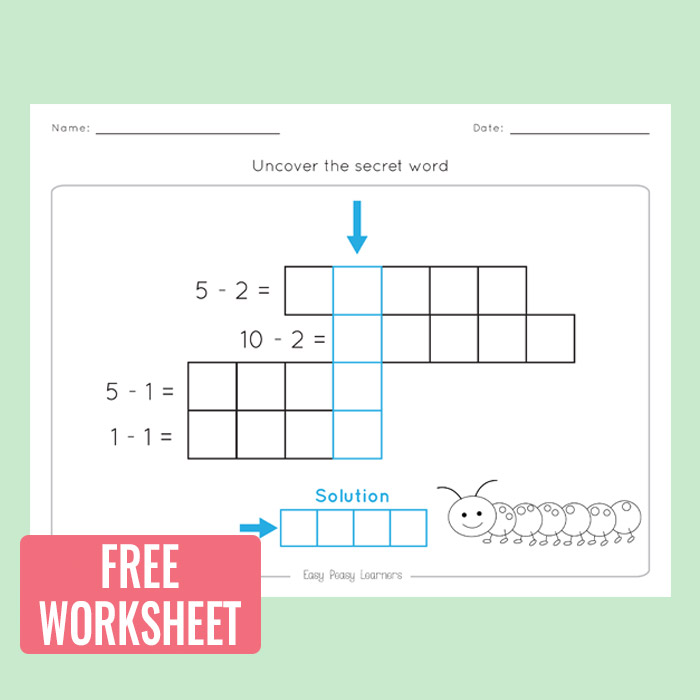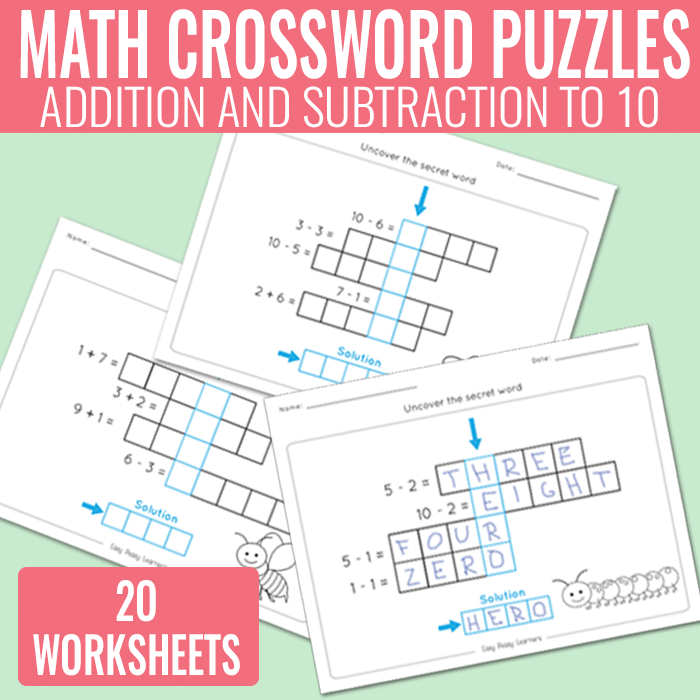# Math Crossword Puzzles Addition and Subtraction to 10 Worksheets

Here is a fun way to practice simple addition and subtraction up to 10, grab this set of math crossword puzzles addition and subtraction to 10 worksheets and let the puzzle solving fun begin.## Math Crossword Puzzles Addition and Subtraction to 10 Worksheets

There are 20 different worksheets in this printable learning pack

• it includes 20 unique addition and subtraction up to 10 worksheets
• students have to solve the equations and write down the answers with words
• each crossword puzzle has a secret word students need to uncover
• pictures on the worksheets are decorative and do not hint to the solution
• there is also an answer key with all the secret word solutions

You can get the whole set at Teachers Pay Teachers where you can also preview the whole set.

Get Adition and Subtraction to 10 Worksheets

Get Addition and Subtraction to 10 Worksheets

These worksheets can be laminated for continuous use.Want to give these a try? You can grab one of the pages for free

Grab the addition and subtraction to 10 freebie worksheet

Get Addition and Subtraction to 10 Worksheets

More Basic Math Learning Resources

Addition to 100 Worksheet – Fun Pyramids to Solve

I Have, Who Has Addition and Subtraction Game

Skip Counting Worksheets (from 2 to 12)